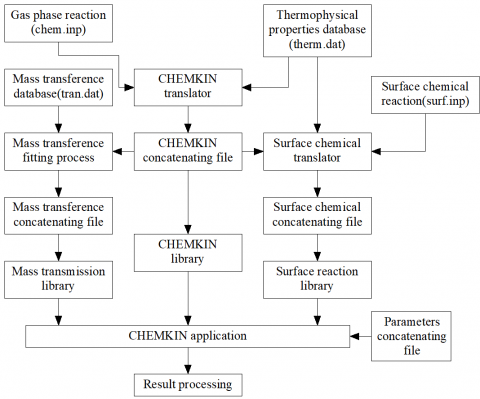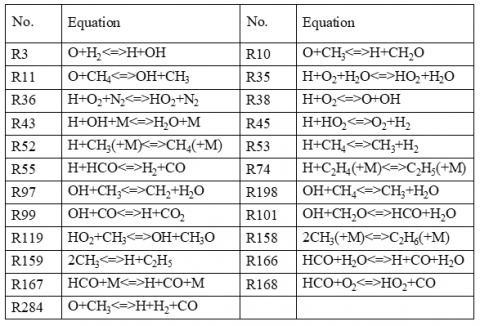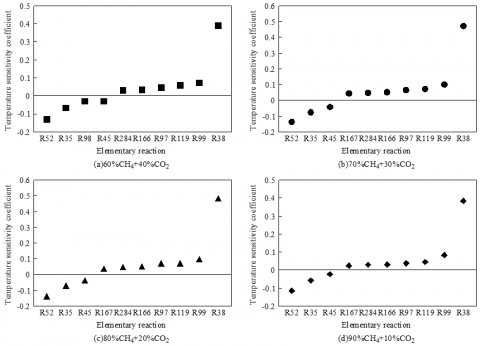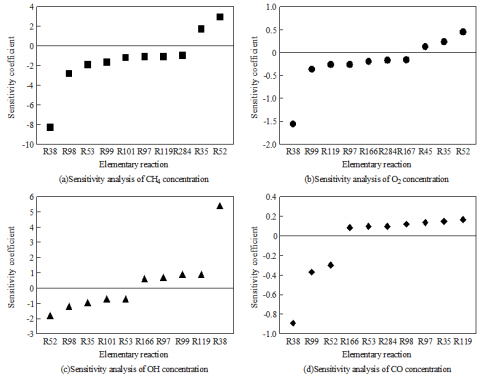# Kinetics Analysis on Chemical Reactions of Hydrocarbon Fuel Based on Computer Simulation

Kinetics Analysis on Chemical Reactions of Hydrocarbon Fuel Based on Computer Simulation

Haijun LiuZhiguo Liu Nan Chen

School of Mechanical and Traffic Engineering, Ordos Institute of Technology, Ordos 017010, China

Hi-TECH Group Corporation, Beijing 100020, China

Dongfeng Commercial Vehicle Technology Center, Wuhan 430070, China

Corresponding Author Email:
lhj_200610@163.com
Page:
117-122
|
DOI:
https://doi.org/10.18280/ijht.370114
19 July 2018
|
Accepted:
5 November 2018
|
Published:
31 March 2019
| Citation

OPEN ACCESS

Abstract:

Hydrocarbon fuel is one of the most heavily used fuels. To optimize the engine performance and realize efficient use of hydrocarbon fuel, it is very meaningful to probe into the combustion mechanism of hydrocarbon fuel in the engine and examine the kinetics principles of the fuel in chemical reactions. In this paper, the chemical kinetics of hydrocarbon fuel was explored with the software CHEMKIN. The author constructed a kinetics model for methane in chemical reactions, and analyzed the main paths of chemical reactions in methane combustion. In addition, the elementary reactions in methane combustion were screened through sensitivity analysis, which focuses on the impacts of the methane concentration, air ratio and combustion products on the sensitivity features of the chemical reaction kinetics model. In this way, the main elementary reactions of methane combustion and the main components of the chemical reaction kinetics model were identified. The comparison between simulated and measured values show that the two values shared a similar trend, indicating that our simplified chemical reaction kinetics model can fulfill the requirements for practical application. The proposed method can be adopted for the numerical simulation of hydrocarbon fuel combustion in the engine.

Keywords:

hydrocarbon fuel, methane, numerical simulation, chemical reaction kinetics

1. Introduction

Hydrocarbon fuel, one of the most heavily used fuels, has been widely adopted as the power fuel for machines and vehicles and the non-power fuel for boilers, catering and daily life [1-2]. An important field of application of hydrocarbon fuel is the engine. To optimize the engine performance and realize efficient use of hydrocarbon fuel, it is very meaningful to probe into the combustion mechanism of hydrocarbon fuel in the engine and examine the kinetics principles of the fuel in chemical reactions [3-4].

Computer numerical simulation is a low-cost technology capable of simulating various complex combustion conditions of hydrocarbon fuel. As a result, this technology has become a popular way to explore the combustion mechanism of hydrocarbon fuel [5-9]. Computer simulation has been frequently employed to construct the kinetics model of hydrocarbon fuel in chemical reactions, aiming to identify the chemical reaction rate of the fuel during combustion and the formation path of combustion products [10-13].

Due to the complex chemical composition of hydrocarbon fuel, multiple movements (e.g. swirl, tumble and turbulence) coexist in the combustion process within the engine. In this case, a full-scale model will be too time-consuming and compute-intensive to be applied in engineering practices [14-15]. To obtain accurate and reliable simulation results, the actual kinetics mechanism of hydrocarbon fuel in chemical reactions must be simplified . The existing simplification methods include principal component analysis (PCA), singular perturbation theory, adaptive list sequential sampling method, Shell model, Hu-Keck model, etc. . All these approaches are based on ideal models, which have many hypotheses and idealized conditions. The computing results deviate far from the actual combustion results of hydrocarbon fuel.

To solve the above defects, this paper creates a kinetics model for methane gas, a typical hydrocarbon fuel, in chemical reactions, and analyzes the effects of methane concentration, air ratio and chemical combustion products on the sensitivity features of the said model. The research conclusions provide a theoretical reference for the numerical simulation of the combustion process of hydrocarbon fuel in the engine.

2. Kinetics Model for Hydrocarbon Fuel in Chemical Reactions

2.1 Introduction to numerical simulation software

The simulation software used in this research is CHEMKIN. As shown in Figure 1, the software mainly consists of data processors, program libraries and reactors. When using CHEMKIN software to calculate the chemical kinetics of hydrocarbon fuel, the user needs to firstly select a specific calculation module, then establish a reaction model of hydrocarbon fuel, pre-process the relevant parameters of the output parameters, solve the reaction model, and finally post-process the output problem.

## figure_1.pngFigure 1. Structure of CHEMKIN

2.2 Chemical reaction kinetics model

The following hypotheses were made before the computer simulation of the combustion of hydrocarbon fuel in the engine:

(1) The basic parameters (e.g. pressure and temperature) are distributed uniformly in the engine;

(2) The thermodynamic parameters are only affected by the type of fuel and the temperature inside the engine;

(3) There is no mass exchange with the outside during the reactions of the hydrocarbon fuel in the combustion chamber.

Under the above hypotheses, the mass change rate of hydrocarbon fuel can be expressed as:

$\frac{\text{d}{{m}_{k}}}{\text{d}t}=V{{\omega }_{k}}{{W}_{k}}$ (1)

According to the first law of thermodynamics, we have:

$\text{d}e+p\text{d}v=0$ (2)

where, P  is the pressure in the combustion chamber; de is the unit internal energy of the material in the combustion chamber. Then, the total internal energy e can be obtained as:

$e=\sum\limits_{k=1}^{K}{{{e}_{k}}{{Y}_{k}}}$ (3)

Differentiating on both sides of the above formula, we have:

$\text{d}e=\sum\limits_{k=1}^{K}{{{Y}_{k}}\text{d}{{e}_{k}}}+\sum\limits_{k=1}^{K}{{{e}_{k}}\text{d}{{Y}_{k}}}$ (4)

Finding the derivative to formula (2), we have:

${{c}_{v}}\frac{\text{d}T}{\text{d}t}+\sum\limits_{k=1}^{K}{{{e}_{k}}\frac{\text{d}{{Y}_{K}}}{\text{d}t}}+p\frac{\text{d}v}{\text{d}t}=0$ (5)

Next, the average specific heat cv and p of the fuel in the engine can be respectively expressed as:

$\begin{matrix} {{c}_{v}}=\sum\limits_{k=1}^{K}{{{Y}_{k}}{{c}_{v,k}}} & p=\frac{\rho RT}{{\bar{W}}} \\ \end{matrix}$ (6)

Substituting formula (6) into formula (5), we have:

$\frac{\text{d}T}{\text{d}t}\sum\limits_{k=1}^{K}{{{Y}_{k}}{{c}_{v,k}}}+\frac{\rho RT}{m\bar{W}}\frac{\text{d}V}{\text{d}t}+v\sum\limits_{k=1}^{K}{{{e}_{k}}{{\omega }_{k}}{{W}_{k}}}=0$ (7)

The chemical reaction rate of the fuel in the engine can be described as:

$\frac{\text{d}Y}{\text{d}t}=\text{f}\left( \text{Y},k \right)\text{ Y}\left( {{t}_{0}} \right)={{\text{Y}}_{0}}$ (8)

Formula (8) can be rewritten as follows after the Taylor series expansion

$y_i (t)=y_i(t,k,Y_0)+\sum(\frac{∂y_i(t)}{∂k_n}(k_n'-k_n)+\sum(\frac{∂y_i(t)}{∂y_m^0})(y_m^(0')-y_m^0)+…$ (9)

where, Y is a variable function with respect to time; k is the parameter of chemical reaction rate. Formulas (8) and (9) make it possible to identify the character parameter that has the greatest impact on fuel combustion under uncertain factors.

2.3 Chemical reaction paths of methane

In this paper, methane, the most basic hydrocarbon fuel, is selected for the analysis on the chemical reaction mechanism in the fuel combustion process. Based on the theories of chemical reaction kinetics, the methane combustion process can be split into three paths:

(1) The methane reacts with oxygen at high temperatures, producing intermediates like CO2, formic acid and formaldehyde.

CH_4 \to CH_3 \begin{align*} & \nearrow {CH_2O \to HCO \to CO \to CO_2}\\ & \searrow {CH_2 \to CH \to C} \end{align*}

(2) The carbon atoms in the methane aggregates into polymers like ethylene and ethane.

$C{{H}_{3}}\to {{C}_{2}}{{H}_{6}}\to {{C}_{2}}{{H}_{5}}\to {{C}_{2}}{{H}_{4}}\to {{C}_{2}}{{H}_{3}}\to C{{H}_{2}}$

(3) The intermediates of methane combustion reacts with N2O and CN, forming NO2 and other final products.

## eq.pngIn actual combustion, the chemical reactions in combustion are affected only by some of the fuel components. Thus, the sensitivity of different components needs to be analyzed before the simulation of fuel combustion, aiming to identify the main influencing components of the reactions and simply the kinetics mechanism of chemical reactions.

3. Test Results and Analysis

To begin with, the author investigated the component sensitivity of the chemical reactions in methane combustion. The elementary reactions in methane combustion are listed in Table 1. Here, different concentrations of methane are mixed with CO2 and subjected to combustion. The temperature sensitivity coefficients of the mixed fuels were measured. The statistical results are shown in Figure 2.

The values in Figure 2 stand for the maximum sensitivity coefficients of the methane-CO2 mixtures in the combustion process. Obviously, R38 and R52 were the main exothermic and endothermic reactions in methane combustion, respectively. The combustion equation of methane can be expressed as:

$C{{H}_{4}}+2{{O}_{2}}=2{{H}_{2}}O+C{{O}_{2}}$

It can be seen from Table 1 that the elementary reactions R38 and R52 were the main reaction process of the methane combustion, indicating that the chemical reaction rate of methane combustion is determined by R38 and R52. According to the Temperature sensitivities of the methane-CO2 mixtures in Figure 2, the intermediate methyl (CH3) of methane production is mainly affected by H free radical.

Table 1. The elementary reactions of methane

## table_1.png## figure_2.pngFigure 2. Temperature sensitivities of the methane-CO2 mixtures

Figure 3 presents the temperature sensitivities of methane at four different excess air ratios. In general, R38 had the highest positive sensitivity under the four air ratios. This is because free radicals O, H, -OH play a major role in methane combustion: these free radicals destroy the macromolecular bonds in the methane, pushing up the thermal decomposition and heat release of methane.

In addition, when methane had a heavy presence in the gas mixture, lots of methyl was produced, and the maximum negative sensitivity appeared in R52; otherwise, few methyl was produced, and the maximum negative sensitivity appeared in R35, which consumed more H than any other elementary reaction.

Figure 4 displays the temperature sensitivities of different chemicals at the air ratio of 1.0. From the CH4 results in Figure 4(a), it is known that the elementary reactions with negative sensitivity, namely, R11, R38, R53 and R97, were the leading influencers of methane concentration. These elementary reactions produced the main free radicals among the intermediaries of methane combustion, and increased the concentration of the active centers. Moreover, R35 and R52 served as elementary reactions with positive sensitivity. Both consumed the free radicals in the chemical products, thus preventing the formation of methane. It can be seen from Figure 4(a) that the elementary reactions with negative sensitivity had much larger sensitivity coefficients than those with positive sensitivity. That is why the mass of the methane combustion system gradually declined in the later stage.

## figure_3.pngFigure 3. The temperature sensitivities of methane at four different excess air ratios

## figure_4.pngFigure 4. The temperature sensitivities of different chemicals at the air ratio of 1.0

As shown in Figure 4(b), the elementary reactions R35, R45 and R52 had positive sensitivity coefficients to O2, and exerted a major impact on the increase of O2 concentration. By contrast, R38 and R99 had negative sensitivity coefficients to O2, i.e. the O2 content will plunge rapidly with the growth in the reaction rates of R38 and R99. Figure 4(b) shows that the positive sensitivity coefficients were greater than the negative ones, which contributes to the gradual reduction of O2 during the combustion process.

As can be seen from Figure 4(c), all elementary reactions were highly sensitive to OH. Among them, R38 (positive sensitivity) and R52 (negative sensitivity) were the top two influencers of OH concentration. The amount of OH free radicals was gradually on the rise, as the sensitivity coefficient of R38 was greater than that of R52.

Figure 4(d) reveals that R38 and R52 significantly promoted the CO consumption. Since the sensitivity coefficients were relatively small, the CO concentration was reduced at a slow rate.

According to above analysis, the combustion reactions of methane were further screened. It is confirmed that elementary reactions R35, R38, R52, R97, R99 and R168 are important parts of methane combustion, while hydrogen, methyl, ethyl, nitrogen, nitric oxide, water, carbon monoxide, carbon dioxide, nitrogen dioxide and free radicals like O, H, N and CH are the main components of the chemical reaction kinetics model for methane combustion.

## figure_5.pngFigure 5. The variations in engine internal pressure, temperature and main products with air ratios

Figure 5 records the variations in engine internal pressure, temperature and main products with air ratios. The solid and dotted curves are respectively the simulated value and the measured value. It can be seen that the trend of the simulated value basically agreed with that of the measured value. Under different air ratios, the simulated engine pressures and temperatures deviated from the measured results by about 2%, while the simulated values of the four main chemical products were basically the same as the measured values. Hence, simplified chemical reaction kinetics model proposed in this paper can fulfill the requirements for practical application. The proposed method can be adopted for the numerical simulation of hydrocarbon fuel combustion in the engine.

4. Conclusions

In light of the combustion features of hydrocarbon fuel, a chemical reaction kinetics model was constructed for methane gas, a typical hydrocarbon fuel. Then, the computer simulation was employed to analyze the impacts of the methane concentration, air ratio and combustion products on the sensitivity features of the chemical reaction kinetics model. The simulated results were compared with the measured results of engine combustion products. The comparison proves the effectiveness of our simplified chemical reaction kinetics model. The research conclusions are as follows:

(1) The chemical kinetics of hydrocarbon fuel was explored with the software CHEMKIN. The author constructed a kinetics model for methane in chemical reactions, and analyzed the main paths of chemical reactions in methane combustion.

(2) The elementary reactions in methane combustion were screened through sensitivity analysis, which focuses on the impacts of the methane concentration, air ratio and combustion products on the sensitivity features of the chemical reaction kinetics model. It is confirmed that elementary reactions R35, R38, R52, R97, R99 and R168 are important parts of methane combustion, while hydrogen, methyl, ethyl, nitrogen, nitric oxide, water, carbon monoxide, carbon dioxide, nitrogen dioxide and free radicals like O, H, N and CH are the main components of the chemical reaction kinetics model for methane combustion.

(3) The comparison between simulated and measured values show that the two values shared a similar trend, indicating that our simplified chemical reaction kinetics model can fulfill the requirements for practical application. The proposed method can be adopted for the numerical simulation of hydrocarbon fuel combustion in the engine.

Acknowledgment

Science and Technology Project of University in Inner Mongolia Autonomous Region (NJZY17405).

References

 Ahmed S, Krumpelt M. (2001). Hydrogen from hydrocarbon fuels for fuel cells. International Journal of Hydrogen Energy 26(4): 291-301. https://doi.org/10.1016/s0360-3199(00)00097-5

 Muradov N, Choi P, Smith F, Bokerman G. (2010). Integration of direct carbon and hydrogen fuel cells for highly efficient power generation from hydrocarbon fuels. Journal of Power Sources 195(4): 1112-1121. https://doi.org/10.1016/j.jpowsour.2009.09.010

 Yadav SPR, Saravanan CG, (2015). Engine characterization study of hydrocarbon fuel derived through recycling of waste transformer oil. Journal of the Energy Institute 88(4): 386-397. https://doi.org/10.1016/j.joei.2014.10.006

 Frolov SM, Aksenov VS, Ivanov VS, Shamshin IO, (2016). Thrust characteristics of a pulse detonation engine operating on a liquid hydrocarbon fuel. Russian Journal of Physical Chemistry B 10(2): 291-297. https://doi.org/10.1134/s1990793116020184

 Banerjee S, Tangko R, Sheen DA, Wang H, Bowman CT, (2016). An experimental and kinetic modeling study of n -dodecane pyrolysis and oxidation. Combustion & Flame 163: 12-30. https://doi.org/10.1016/j.combustflame.2015.08.005

 Montgomery CJ, Cremer, MA, Chen JY, Berkeley B, Westbrook CK, Maurice LQ. (2012). Reduced chemical kinetic mechanisms for hydrocarbon fuels. Journal of Propulsion & Power 18(1): 192-198. https://doi.org/10.2514/2.5916

 Garg R, Thakur H, Tripathi B. (2018). Numerical simulation of two-dimensional fluid flow problem using truly meshfree method. Mathematical Modelling of Engineering Problems 5(4): 357-364. https://doi.org/10.18280/mmep.050412

 Petrova MV, Williams FA. (2006). A small detailed chemical-kinetic mechanism for hydrocarbon combustion. Combustion & Flame 144(3): 526-544. https://doi.org/10.1016/j.combustflame.2005.07.016

 Zhang W, Yu YD, Li Z, Li JP. (2018). Study on closed-die forging technology and numerical simulation of T-junction of high-pressure pipe. Revue des Composites et des Matériaux Avancés 28(3): 439-448. https://doi.org/10.3166/RCMA.28.439-448

 You X, Egolfopoulos FN, Wang H. (2009). Detailed and simplified kinetic models of n -dodecane oxidation: the role of fuel cracking in aliphatic hydrocarbon combustion. Proceedings of the Combustion Institute 32(1): 403-410. https://doi.org/10.1016/j.proci.2008.06.041

 Westbrook CK. (2015). Chemical kinetic modeling of higher hydrocarbon fuels. Aiaa Journal 24(12): 2002-2009. https://doi.org/10.2514/3.9559

 Ranzi E, Dente M, Goldaniga A, Bozzano G, Faravelli T. (2001). Lumping procedures in detailed kinetic modeling of gasification, pyrolysis, partial oxidation and combustion of hydrocarbon mixtures. Progress in Energy & Combustion Science 27(1): 99-139. https://doi.org/10.1016/s0360-1285(00)00013-7

 Simmie JM. (2003). Detailed chemical kinetic models for the combustion of hydrocarbon fuels. Progress in Energy & Combustion Science 29(6): 599-634. https://doi.org/10.1016/s0360-1285(03)00060-1

 Westbrook CK. (2000). Chemical kinetics of hydrocarbon ignition in practical combustion systems. Proceedings of the Combustion Institute 28(2): 1563-1577. https://doi.org/10.1016/s0082-0784(00)80554-8

 Volchkov ÉP, Dvornikov NA. (2006). Modeling of incomplete combustion of hydrocarbons in the presence of water under high pressure. Combustion Explosion & Shock Waves 42(3): 277-281. https://doi.org/10.1007/s10573-006-0051-5

 Luo Z. (2013). Development of reduced chemical kinetics for combustion simulations with transportation fuels. Computer Engineering & Applications 46 (8): 56-58.

 Adžić M, Fotev V, Jovičić V, Milivojević A, Milekić G, Adžić V, Bogner M. (2008). Potentials for usage of significantly reduced chemical mechanisms in numerical modeling of combustion processes. Fme Transactions 36(1): 1-8.